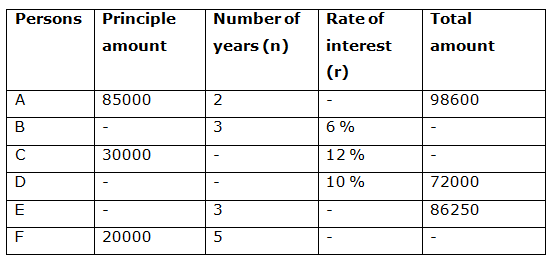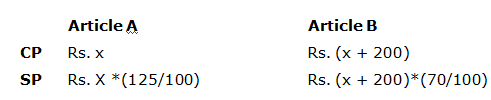# IBPS PO Mains 2018 – Quantitative Aptitude Questions Day-12

Dear Readers, Bank Exam Race for the Year 2018 is already started, To enrich your preparation here we have providing new series of Practice Questions on Quantitative Aptitude – Section. Candidates those who are preparing for IBPS PO Mains 2018 Exams can practice these questions daily and make your preparation effective.

[WpProQuiz 4092]

Directions (Q. 1 – 5): Each question contains Quantity I and Quantity II. Read the contents clearly and answer your questions accordingly.

1) Quantity I: The length of the rectangle is twice the side of a square whose area is 484 Sq. m. The breadth of the rectangle is 15 m less than the length of the rectangle. Find the area of rectangle?

Quantity II: The perimeter of a rectangle is 176 m. The difference between the length and breadth of the rectangle is 16 m. Find the area of a rectangle?

a) Quantity I > Quantity II
b) Quantity I ≥ Quantity II
c) Quantity II > Quantity I
d) Quantity II ≥ Quantity I
e) Quantity I = Quantity II or Relation cannot be established

2) Quantity I: The ratio between the downstream and upstream speed of the boat is 3: 2. The boat travels 72 km downstream in 8 hours. Find the speed of the boat in still water?

Quantity II: The boat travels 90 km downstream in 6 hours. The speed of the stream is 6 km/hr. Find the speed of the boat in still water?

a) Quantity I > Quantity II
b) Quantity I ≥ Quantity II
c) Quantity II > Quantity I
d) Quantity II ≥ Quantity I
e) Quantity I = Quantity II or Relation cannot be established

3) Pragathi bought two articles A and B at Rs. X and Rs. X + 200 respectively. She sold article A at 25 % profit and article B at 30 % loss, and he loss Rs. 110 on the whole deal.

Quantity I:  Find the profit earned (In Rs.) by Pragathi on selling article A?

Quantity II: Find the loss incurred (in Rs.) by Pragathi on selling Article B?

a) Quantity I > Quantity II
b) Quantity I ≥ Quantity II
c) Quantity II > Quantity I
d) Quantity II ≥ Quantity I
e) Quantity I = Quantity II or Relation cannot be established

4) A box contains 6 Red balls, 4 white balls, 5 orange balls and 7 black balls.

Quantity I: If three balls are drawn at random, then find the probability of getting at least one black ball?

Quantity II: If four balls are drawn at random, then find the probability that all are of different colours?

a) Quantity I > Quantity II
b) Quantity I ≥ Quantity II
c) Quantity II > Quantity I
d) Quantity II ≥ Quantity I
e) Quantity I = Quantity II or Relation cannot be established

5) Quantity I: If ∛512 x – √9216 = 0, then find the value of x?

Quantity II: If x/(2x + y) = 4/13, then find the value of y?

a) Quantity I > Quantity II
b) Quantity I ≥ Quantity II
c) Quantity II > Quantity I
d) Quantity II ≥ Quantity I
e) Quantity I = Quantity II or Relation cannot be established

Directions (Q. 6 – 10): Study the following information carefully and answer the given questions.

The following table shows the principle amount invested by 6 different persons, the number of years and rate of simple interest per annum and total amount received by them after completing the certain time period. Some values are missing here. We have to find the answer according to the question given.6) Find the difference between the interest received by the person D to that of person E, if the ratio between the rate of interest of person D to that of E is 2 : 1 and the period of investment is same for both the person A and D?

a) Rs. 1500

b) Rs. 2300

c) Rs. 750

d) Rs. 1850

e) None of these

7) Find the ratio between the interest received by the person B to that of C, if the total amount received by the person B after completing the given period is Rs. 47200 and the total amount received by the person C is Rs. 4900 less than the half the amount received by A?

a) 1: 2

b) 3: 4

c) 2: 3

d) 4: 5

e) None of these

8) Find the principle amount invested by D, if the average principle amount invested by all the given persons except E is Rs. 47000 and the principle amount invested by B is 20000 more than D?

a) Rs. 50000

b) Rs. 25000

c) Rs. 60000

d) Rs. 40000

e) None of these

9) The rate of interest for the person A is what percentage of the rate of interest for the person F, if the interest received by F is Rs. 15000?

a) 42.25 %

b) 53.33 %

c) 58.5 %

d) 63.67 %

e) None of these

10) Find the average total amount received by the person B, D and F together, if the principle amount invested by B is Rs. 40000 and the total interest received by F is Rs. 15000?

a) Rs. 53500

b) Rs. 58200

c) Rs. 51400

d) Rs. 49700

e) None of these

Direction (1-5) :

Quantity I:

Area of the square = a2 = 484

Side of the square (a) = 22 m

Length of the rectangle = 44 m

Area of the rectangle = 44*29 = 1276 Sq. m

Quantity II:

The perimeter of a rectangle = 176 m

2*(l + b) = 176

l + b = 88 —à (1)

l – b = 16 —à (2)

By solving the equation (1) and (2), we get,

Length = 52 m, Breadth = 36 m

Area of the rectangle = 52*36 = 1872 Sq. m

Quantity II > Quantity I

Quantity I:

Downstream speed= 72/8 = 9 km/hr

Upstream speed= 9*(2/3) = 6 km/hr

Speed of boat in still water = ½ *(9+6) = 7.5 km/hr

Quantity II:

Downstream speed = 90/6 = 15 km/hr

Downstream speed = Speed of boat in still water + speed of stream

Let the speed of boat be x,

=> x + 6 = 15

=> x = 9 km/hr

Speed of boat in still water = 9 km/hr

Quantity I < Quantity IINow, Loss = CP – SP

110 = x + x + 200 – 125x/100 – (x + 200)*(70/100)

110 = 2x + 200 – 125x/100 – 70x/100 – 140

110 = 2x + 60 – 195x/100

50 = (200x – 195x)/100

50 = 5x/100

X = 5000/5 = Rs. 1000

Quantity I:

Profit earned on selling article A

= > 1000*(25/100) = Rs. 250

Quantity II:

The loss incurred on selling article B

= > 1200*(30/100) = Rs. 360

Quantity II > Quantity I

Total number of balls = 6 + 4 + 5 + 7 = 22

Quantity I:

n(S) = 22C3

The probability of getting at least one black ball = 1 – P(none is black ball)

P(none is black ball)

n(E) = 15C3

P(E) = n(E)/n(S) = 15C3/22C3 = 13/44

The probability of getting at least one black ball = 1 – (13/44) = 31/44

Quantity II:

n(S) = 22C4

n(E) = Probability that all the balls are of different colours = 6C1 and 4C1 and 5C1 and 7C1

P(E) = n(E)/n(S) = 24/209

Quantity I > Quantity II

Quantity I:

∛512 x – √9216 = 0

8x = 96

X = 12

Quantity II:

x/(2x + y) = 4/13

12/(24 + y) = 4/13

24 + y = 39

Y = 39 – 24 = 15

Quantity II > Quantity I

Direction (6-10) :

The ratio between the rate of interest of person D to that of E = 2: 1

The rate of interest of person E = (10/2)*1 = 5 %

The period of investment is same for both the person A and D, i.e.,

Period for D = 2 years

Let the principle amount invested by the person D and E be x and y respectively,

The principle amount invested by the person D

= > (x*2*10)/100 = 72000 – x

= > 20x = 7200000 – 100x

= > 120x = 7200000

= > x = (7200000/120) = Rs. 60000

The interest received by the person D

= > (60000*2*10)/100

= > Rs. 12000

The principle amount invested by the person E

= > (y*3*5)/100 = 86250 – y

= > 15y = 8625000 – 100y

= > 115y = 8625000

= > y = 8625000/115 = Rs. 75000

The interest received by the person E

= > (75000*3*5)/100 = Rs. 11250

Required difference = 12000 – 11250 = Rs. 750

The total amount received by the person B after completing the given period

= > Rs. 47200

The total amount received by the person C

= > (98600/2) – 4900

= > Rs. 44400

Let the principle amount invested by B be x,

The principle amount invested by the person B

= > (x*3*6)/100 = 47200 – x

= > 18x = 4720000 – 100x

= > 118x = 4720000

= > x = 4720000/118 = Rs. 40000

The interest received by the person B

= > (40000*3*6)/100 = Rs. 7200

The interest received by the person C

= > 44400 – 30000 = Rs. 14400

Required ratio = 7200: 14400 = 1: 2

The average principle amount invested by all the given persons except E

= > Rs. 47000

The total principle amount invested by all the given persons except E

= > 47000*5 = Rs. 235000

The principle amount invested by the person B and D

= > 235000 – (85000 + 30000 + 20000)

= > Rs. 100000

B + D = 100000 -à (1)

B – D = 20000 -à (2)

By solving the equation (1) and (2), we get,

2B = 120000

B = 60000 and D = 40000

The principle amount invested by D = Rs. 40000

The rate of interest for the person A

= > (85000*2*r1)/100 = 98600 – 85000

= > r1 = (13600*100)/(85000*2) = 8 %

The interest received by F = Rs. 15000

The rate of interest for the person F

= > (20000*5*r2)/100 = 15000

= > r2 = 1500000/100000 = 15 %

Required % = (8/15)*100 = 53.33 %

The principle amount invested by B = Rs. 40000

The interest earned by the person B

= > (40000*3*6)/100 = Rs. 7200

The total amount received by the person B = 40000 + 7200 = Rs. 47200

The total interest received by the person F = Rs. 15000

The total amount received by the person F = 20000 + 15000 = Rs. 35000

The average total amount received by the person B, D and F together

= > (47200 + 72000 + 35000)/3 = Rs. 51400

Daily Practice Test Schedule | Good Luck

 Topic Daily Publishing Time Daily News Papers & Editorials 8.00 AM Current Affairs Quiz 9.00 AM Current Affairs Quiz (Hindi) 9.30 AM IBPS PO Mains – Reasoning 10.00 AM IBPS PO Mains– Quantitative Aptitude 11.00 PM Vocabulary (Based on The Hindu) 12.00 PM IBPS PO Mains – English Language 1.00 PM IBPS PO/Clerk – GK 3.00 PM Daily Current Affairs Updates 5.00 PM IBPS Clerk Prelims – Reasoning 6.00 PM IBPS Clerk Prelims – Reasoning (Hindi) 6.30 PM IBPS Clerk Prelims – Quantitative Aptitude 7.00 PM IBPS Clerk Prelims – Quantitative Aptitude (Hindi) 7.30 PM IBPS Clerk Prelims – English Language 8.00 PM